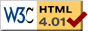### Journée-séminaire de combinatoire

#### (équipe CALIN du LIPN, université Paris-Nord, Villetaneuse)

Le 06 décembre 2011 à 14h00 en B311, Luciano Norberto Grippo nous parlera de : Forbidden subgraph characterizations of some classes of intersection graphs

Résumé : Given a finite family of non-empty sets F. The intersection graph of F is obtained by representing each set by a vertex, two vertices being adjacent if and only if their corresponding sets intersect. In this talk we will focus on two important intersection graph classes, namely circular-arc graphs (intersection graphs of arc on a circle) and circle graphs (intersection graphs of chords on a circle). We will present some partial characterizations by forbidden induced subgraphs for these classes. Let F be a family of graphs. A graph $G$ is said to be a probe--F graph if its vertex set can be partitioned into two sets: a set P of probe vertices and a stable set N of non-probe vertices in such a way that edges, whose endpoints belongs to N, can be added to G so that the resulting graph belongs to F. We will present some forbidden induced subgraphs characterizations for probe interval graphs and probe unit interval graphs on superclasses of cographs. Finally, we will present a complete forbidden induced subgraphs characterization of probe block graphs. This talk is based on a mix of joint works with F. Bonomo, G. Durán and M. Safe.

 Dernière modification : Monday 24 January 2022Contact pour cette page : Cyril.Banderier at lipn.univ-paris13.fr